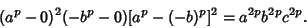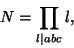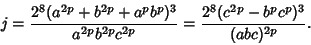## Frey Curve

Letbe a solution to Fermat's Last Theorem. Then the corresponding Frey curve is(1)

Frey showed that such curves cannot be Modular, so if the Taniyama-Shimura Conjecture were true, Frey curves couldn't exist and Fermat's Last Theorem would follow withEven and. Frey curves are Semistable. Invariants include the Discriminant(2)

The Minimal Discriminant is(3)

the Conductor is(4)

and the j-Invariant is(5)

Cox, D. A. Introduction to Fermat's Last Theorem.'' Amer. Math. Monthly 101, 3-14, 1994.
Gouvêa, F. Q. A Marvelous Proof.'' Amer. Math. Monthly 101, 203-222, 1994.